Impact Factor 2.638 | CiteScore 2.3
More on impact ›

# Frontiers in Physics## Original Research ARTICLE

Front. Phys., 13 November 2020 | https://doi.org/10.3389/fphy.2020.585590

# Steady-State 3D Trapping and Manipulation of Microbubbles Using Thermocapillary

• 1Optical Fiber Laboratory, Universidad Politécnica de Tulancingo, División de Posgrado, Tulancingo, México
• 2Instituto Nacional de Astrofísica, Óptica y Electrónica, Departamento de Óptica, Puebla, México

An experimental and theoretical study on the 3D trapping and manipulation of microbubbles by means low power laser-induced temperature gradients induced in ethanol by bulk light absorption (λ = 1550 nm) is presented. Two optical fibers were used: One for bubble generation (OFG) and the other for both trapping and manipulation (OFT). Light from a Q-switched pulsed laser (λ = 532 nm and pulse width τp = 5 ns) propagates in fiber OFG and gets absorbed at silver nanoparticles (AgNPs), previously photodeposited, at the distal end of a fiber optic core, generating the microbubbles. In the fiber OFT, light of low power CW laser was used to trap and manipulate the bubbles by thermocapillary induced by light bulk absorption in ethanol. The microbubble generated on OFG migrates toward the fiber OFT. The equilibrium between the buoyancy force FB, drag force FD and the Marangoni force (also known as thermocapillary force) FM gives rise to a 3D stably trapping and manipulation of the microbubble for the best time to our best knowledge.

## Introduction

Trapping and manipulation of microbubbles have become a very useful tool in various applications such as manipulation of micro-objects [1, 2], fabrication of micro-valves [3, 4], photolithography , among others. There are different techniques for generating, trapping, and manipulating microbubbles in liquids using thermal , acoustic [9, 10], and optical effects . Thermal effects have been considered as an unwanted side effect on optical trapping; however, forces of thermal origin are orders of magnitude greater than optical ones, thus offering plenty of opportunities for the manipulation of micro-objects [1, 2]. In fact, generation and 2D trapping of microbubbles using light induced temperature gradient using absorbent thin films deposited on one of the substrates has been demonstrated for several authors [5, 14, 15]. Later, absorption in the bulk and from nano/microparticles suspended on the liquid were used to achieve thermal trapping and manipulation [8, 16, 17]. More recently, Benerjee et al. , reported the trapping and 2D manipulation of bubbles due themal bluming and Marangoni effect triggered by light absorption of a focused CW laser on colloidal particles suspended in isopropanol. However, they require rather large optical powers >100 mW; besides, they did neither show steady-state trapping nor stably manipulation. Recently, our research group has demonstrated both the generation and quasi-steady-state trapping and manipulation of single microbubbles in optical fibers using the Marangoni effect [19, 20].

Here, we report the 3D trapping and manipulation of a microbubble through temperature gradients generated by light absorption. Two optical fibers were used: One for bubble generation (OFG) and the other for trapping and manipulation (OFT). In the fiber OFG, light from a Q-switched pulsed laser propagates and gets absorbed at silver nanoparticles, previously photodeposited at the distal end of the fiber optic core, generating the microbubbles. In the second fiber OFT, the light from a low power CW laser is used to trap and manipulate the bubbles by Marangoni force induced by light absorption in ethanol. The generated microbubble on fiber OFG migrates toward the fiber OFT. The equilibrium trapping position around the fiber OFT is determined by the balance between the buoyancy force (FB), drag force (FD), and the Marangoni force, also known as thermocapillary force (FM). To our best knowledge, this is the first time that 3D stable trapping and manipulation of the microbubble in liquids is reported.

## Experimental Section

A beam from a pulsed second harmonic laser (λ = 532 nm, τp = 5 ns, Spectra-Physics Q-switching Mod. Explorer 532–200-E) is coupled into a multimode optical fiber (OFG, 50/125 µm), using an aspherical lens with a focal distance of 4.5 mm as shown in Figure 1. Previously, AgNPs were immobilized using the photodeposition technique at the distal end of the fiber OFG . The optical power loss caused by AgNPs absorption was approximately 2 dB. When light impinges on the AgNPs, they are heated up well beyond the ethanol’s boiling temperature leading to the creation of thermocavitation bubbles . A second laser, continuous wave (CW) laser (λ = 1,550 nm, Thorlabs model SFL1550S, and current controller model CDL1015) with single-mode optical fiber output (OFT, 9/125 µm) was used for trapping and manipulation of the microbubbles generated at fiber OFG. No nanoparticles were photodeposited on fiber OFT; thermal effects were generated by light absorption in the bulk ethanol (ethanol absorption coefficient at λ = 1,550 nm is $\alpha$ ∼ 5.63 cm−1 or ∼166 µm penetration length ). The fibers OFG and OFT were placed in different configurations inside a 3 ml plastic cell. The visualization of the generation, trapping, and manipulation was done through a 5× microscope objective (Newport M-5X), a white LED, and a Motic3 camera (3 Mpx resolution) connected to a CPU.

FIGURE 1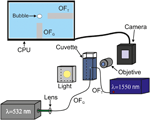FIGURE 1. Experimental set up for generation and trapping of microbubbles. The microbubbles are generated at fiber OFG using a pulsed laser (λ = 532 nm, τp = 5 ns) and trapped at fiber OFT using a CW low power laser (λ = 1550 nm, Power 1–15 mW).

## Experimental Results

Figure 2 shows the generation and trapping of a microbubble for different fiber OFT positions (fiber end facing downwards, horizontally, and upwards). The AgNPs strongly absorb light from the laser at 532 nm increasing its temperature. By heat transfer, the surrounding liquid is heated up well beyond its boiling temperature and eventually, evaporates explosively creating a microbubble that is expelled from the fiber end . The longer the pulsed laser is on, the larger the bubble's diameter . In particular, the radius reached by the microbubble was approximately R ∼ 42 µm for all the cases shown in Figure 2. The microbubble ascends through the fluid due to buoyancy, and in minor scale by convective currents, as shown in Figures 2B,E,H. When the bubble leaves the fiber OFG, the CW laser (λ = 1,550 nm) is turned on heating up the liquid along its propagation path generating a temperature gradient attracting the microbubble toward it. This region is located along the propagation axis of the fiber OFT, when the forces (Marangoni, drag, and buoyancy) are in equilibrium (see Figures 2C,F,I) then the microbubble becomes trapped. The separation distance d between the fiber end OFT and the center of the microbubble when the fiber OFT was facing downwards, horizontally, and upwards was: ∼75, ∼100, and ∼350 μm, respectively.

FIGURE 2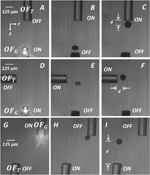FIGURE 2. Generation and 3D trapping of a microbubble for different fiber OFT positions: facing downwards 2A, horizontally 2D, and upwards 2G. Bubble release from the fiber OFG: 2B, 2E, and 2H. Bubble trapping 2C, 2F, and 2I. See visualizations 1, 2, and 3 for more details.

The 3D manipulation of a microbubble when the fiber end OFT was facing downwards, horizontally, and upwards can be seen in visualizations 1, 2, and 3, respectively. For each of the cases shown in visualizations, the radius of the microbubble was R ∼ 42 µm. Note that the bubble follows the fiber OFT displacements since the temperature gradient moves along with it. When the fiber OFT is pointing upwards the microbubble is trapped at a greater distance d. Trapping is possible for different microbubble radii, as shown in Figure 3.

FIGURE 3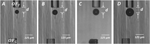FIGURE 3. Trapping of microbubbles through a temperature gradient induced with a power of 2 mW for different radii R and its corresponding trapping distance d: (A)R ∼ 32 µm and d ∼ 71 μm, (B)R ∼ 42 and d ∼ 76 μm, (C)R ∼ 50 µm and d ∼ 74 µm, and (D)R ∼ 62 µm and d ∼ 78 µm.

Microbubbles of radius R ≥ 130 µm get in contact with the fiber OFT, as one can see in Figures 4A,B. For these large bubbles, trapping becomes unstable and manipulation is not possible. Thus, a good criterion for an upper limit of bubble manipulation is to choose those bubbles whose diameter is comparable with the OFT’s diameter. On the other hand, the lower limit of trapped bubbles size could not determine since bubbles with diameter ≤30 µm are very difficult to create as they growths very fast (≤100 ms) which comparable to the response time of our mechanical shutter. However, with the proper shutter, the laser could be turned on from µs to ms and, thus, obtaining smaller bubbles.

FIGURE 4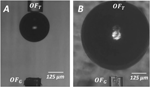FIGURE 4. Trapping of microbubbles with a power of 2 mW. Large bubbles touch the optical fiber OFT(A)R ∼ 130 μm, and (B)R ∼ 333 µm. For microbubbles of radius R ≥ 130 µm trapping becomes unstable and manipulation is not possible.

Polystyrene microparticles (diameter ∼ 1 µm) were dispersed in ethanol to be used as tracers to measure the velocity of the convective currents induced by light absorption with a power of 2 mW. Figure 5 shows the tracking of a cluster of microparticles due to the convective currents and the obtained velocity of these currents along the propagation axis. By video analysis, the velocity of the convective currents around of the optical fiber end (z = 120 µm) was found to be ∼0.64 mm/s in the upward direction. For the largest used power in this work (15 mW) and for the same region (z = 120 µm), the speed scales almost linearly ∼7.7 mm/s.

FIGURE 5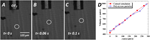FIGURE 5. (A–C) Snapshots show that particles move in opposite direction to the beam propagation at a laser power p = 2 mW. White circles indicate the position of a cluster of several polystyrene microparticles. (D) Profile of the convective currents’ velocity as a function of propagation distance z measured from the fiber end. Blue dots correspond to the microparticles' velocity whereas red squares correspond to the convective currents obtained from the Comsol simulation.

## Discussion

Experimental results show the trapping and manipulation of microbubbles, previously generated, using optically-induced temperature gradients caused by light absorption in ethanol . Microbubbles are generated in ethanol by thermocavitation, i.e., the explosive phase transition from liquid to vapor around its critical-point (243°C)  after light from a pulsed laser is absorbed at AgNPs deposited at the end of an optical fiber. One key characteristic of thermocavitation is that bubbles remain in contact with the interface (in this case fiber end with photodeposited AgNPs) at all times. Upon collapse, the bubble takes a toroidal shape due to a reentrant jet that eventually hits the hot surface and is instantaneously evaporated . This vapor microbubble is expelled from the fiber end with an exponentially decaying velocity as they move away from the fiber. The repetition rate of the laser is 10 kHz, so every 100 µs, a microbubble is expelled from the fiber creating a column of bubbles moving away from the fiber. Since the bubble velocity is continually decreasing, they eventually catch up and coalesce creating a larger continuously growing bubble. Thus, microbubble size, from tens to hundreds of micrometers in diameter, can be precisely generated by controlling the on-time of the laser (or equivalently, the number of pulses) . This continuously growing bubble can be temporally trapped by the same fiber OFG until it reaches a size such that buoyancy force overcomes the Marangoni force and the bubble leaves the trap. This temporarily trapping last only 55 s. In this work, this bubble is released by turning the pulsed laser off after the bubble reached a certain size and later trapped using another optical fiber with no nanoparticles deposited. This time, the trapping laser is a CW low power laser. The microbubble can be steadily trapped and manipulated for up to10 min. In order to achieve this goal, switch on/off synchronization between the lasers is necessary.

In order to understand the trapping mechanism, we analyze the involved forces using COMSOL Multiphysics 5.2 simulations. Light from the CW laser (λ = 1550 nm) is exponentially attenuated ($\alpha$ ∼ 5.63 cm−1) inside the ethanol, generating a transversal and longitudinal temperature gradient. Without loss of generalization, we will assume that the trapping fiber is pointing downwards so the microbubble will move up toward the trapping fiber by buoyancy force FB. When it is in close proximity to the temperature gradient, it will experience the Marangoni force FM. Due to the temperature gradient, convective currents are also generated within the fluid creating an additional drag force FD. When the microbubble is trapped, an equilibrium between the buoyancy FB, drag FD, and Marangoni FM forces is established. The direction of Marangoni's force is always directed toward the heat source while the buoyancy force and drag force, always points upwards. The equilibrium position is located at a distance d, measured from the tip of the fiber OFT until the bubble geometrical center. Optical forces are not taken into account because their magnitudes are three orders of magnitude smaller than the buoyancy force and six orders of magnitude smaller than the Marangoni force FM .

When the light is incident upon the ethanol a portion of it is absorbed by the ethanol, producing a temperature gradient ∇T that heats the ethanol up according to the heat transfer equation (where a steady-state condition is assumed) is given by :

$ρCpu⋅∇T=∇⋅(k∇T)+Q,(1)$

where ρ is the ethanol density, Cp is the heat capacity, u is the fluid's field velocity, k is the thermal conductivity, and Q = αI is the heat source per volume unit with α the absorption coefficient and I the optical intensity of the Gaussian beam. To model the fluid's field velocity, both the heat transfer equation given by Eq. 1 and the Navier-Stokes equations for incompressible fluids, given by Eqs 2 and 3, are solved by finite element method (Comsol Multiphysics).

$ρ(u⋅∇)u=∇⋅[−pH+μ(∇u+(∇u)T)]+F,(2)$
$ρ∇⋅u=0,(3)$

where H is the identity matrix, µ is the ethanol viscosity, and F is the volumetric force per volume unit defined as F = g(ρρ0) where g is the gravitational acceleration, ρ is the ethanol density at temperature T, and ρ0 = 789 kg/m3 is the ethanol density at room temperature.

The simulation was carried out assuming a 2D configuration given the geometry of the problem, non-slip boundary condition, and initial room temperature in all boundaries. We use an inhomogeneous mesh been finer in the vicinity of the optical fiber (element size = 1.5 µm) in order to reduce the computing time. We considered a Gaussian laser beam whose spot corresponds to the fiber core radius ${\omega }_{0}=4.5\text{ μm}$, the absorption coefficient of ethanol of, a cuvette with square geometry of width 1 mm, and height 5 mm. The origin of the coordinate system is set at the optical fiber end. The cuvette used in the experiments is much larger than the cuvette used in the simulation but the results (temperature and liquid's velocity) do not change much (∼2%) respect to real cuvette. We prefer to keep the small container for the sake of computational time.

Figure 1 shows the spatial temperature distribution induced by a 2 mW power laser. As expected, the temperature increases as it travels inside the liquid, reaches a peak, and eventually decreases exponentially according to Beer-Lambert law to room temperature for z2 mm. Figure 6B shows the temperature distribution along the propagation distance for different optical powers showing the same general behavior. Note the abrupt rise of the temperature from room temperature until the peak one which occurs at a distance zHT ∼ 75.4 µm independently of the laser power (but determined by the absorption coefficient). Note that the temperature increases linearly with the power from ∼3.4 K for 2 mW to ∼ 26.8 K for the highest power of 15 mW. Figure 6C show the transverse temperature profile at the highest temperature (i.e. zHT = 75.4 µm) with a spatial profile much wider than the Gaussian beam one as consequence of heat diffusion. Finally, Figure 6D shows the velocity of the convective currents generated within the ethanol with an optical power of 2 mW. The convective currents peak velocity is about 0.7 mm/s (around z ∼ 440 µm) in concordance with the measured velocity (seeFigure 7). The associated drag force FD exerted on the bubble is given by:

$FD=6πμRu,(4)$

where $\mu =1.17×{10}^{-3}\text{ Pa}\cdot \text{s}$ . For an optical power of 2 mW, the peak drag force is ∼0.1 nN, i.e., an order of magnitude smaller than the buoyancy force. However, when the highest power is used (15 mW), the drag force is comparable to the buoyancy force and cannot longer be neglected.

FIGURE 6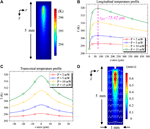FIGURE 6. (A) Temperature distribution on ethanol due to light absorption for a power of 2 mW, (B) longitudinal temperature profile on the propagation axis z for several powers, (C) transverse temperature profile on zTH = 75.4 µm, and (D) convective currents velocity produced by the temperature gradient with an optical power of 2 mW.

As Figure 7A shows, the longitudinal temperature gradient profile obtained from COMSOL Multiphysics is quite steep before ∼75.4 µm and after this point, the gradient is small but sufficiently large as to attract the bubble to the fiber. Note that all temperature gradients change sign at the same distance zHT ∼ 75.4 µm as shown in Figure 8B. The temperature gradient shows great similarity to the optical gradient present in optical traps. In fact, the transversal and longitudinal temperature gradient result in a Marangoni force that traps the bubble in three dimensions, just as in optical trapping.

FIGURE 7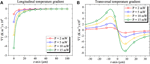FIGURE 7. (A) Longitudinal gradient temperature profile along the propagation axis z and (B) transversal gradient temperature profile obtained at zHT ∼ 75.4 µm, for 2, 5, 10, and 15 mW obtained through simulation in COMSOL Multiphysics.

In the Marangoni force, a tangential stress on the bubble's wall owing to the temperature dependence of the surface tension is exerted; the bubble will move toward the heat source while the liquid flows to the colder regions with a force given by [19, 27]:

$FM=−2πR2∇TdσdT,(5)$

where $\frac{d\sigma }{dT}$ is the temperature derivative of the surface tension of the liquid σ (−0.022 Nm−1) . The buoyancy force ${\mathbf{F}}_{\mathbf{B}}$ which is given by:

$FB=43πρ0gR3,(6)$

where ${\rho }_{0}=789{\text{kg/m}}^{3}$ is the density of the liquid (ethanol) . The total force ${\mathbf{F}}_{\mathbf{T}}$ experienced by a trapped microbubble used in this study is:

$FT=±FM+FB∓FD,(7)$

where the ± sign indicates if the fiber is pointing upwards or downwards, respectively. From Figure 7B and Eq. 5, we know that the bubble will be trapped transversally around r = 0, so lets analyze where the equilibrium position along z is located. Figure 7 shows the longitudinal total force. As expected, the Marangoni force is predominantly close to the optical fiber end where the temperature gradient is larger. When the total force FT on the microbubble is equal to zero the microbubble will be trapped. The total longitudinal force for an optical power of 2 mW and microbubbles radius of 32, 42, 50, and 62 µm is zero at z ∼ 77, 73, 70.5, and 68 μm, respectively (see Figure 8) which are very close to those measured (71–78 µm obtained from Figure 3). As the power increases, the contribution of the drag force becomes comparable to the buoyance force and therefore the trapping distance decreases. One possible explanation for the disagreement between theory and experiment, rely on the fact that our simulation did not include the presence of the bubble. In addition, when the microbubble interacts with the beam laser there is an additional temperature profile that appears on the opposite (exit) surface of the microbubble that modifies the net temperature gradient. Since the Rayleigh distance for the trapping beam is ∼41 μm, as the beam diffracts, the additional thermal gradient is stronger for smaller than larger bubbles (as seen in Figure 2). Nevertheless, this simple model explains reasonably well all the experiments reported.

FIGURE 8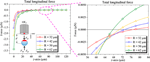FIGURE 8. Total longitudinal force using Eq. 7 for microbubble with radii ∼32, ∼42, ∼50, and ∼62 µm. The zero crossing displaces to the smaller distances z as the convective current increases as discussed in the main text.

In our previous work, we reported quasi-steady-state trapping for milliseconds up to 55 s before the bubble become so large that buoyancy dominates over all forces escaping the trap . Here using CW low power laser, we can extend the trapping time depending on the power used. For example, if a microbubble is trapped with a power 1–2 mW, the rate of vapor condensation exceeds that of evaporation and therefore the bubble will shrink over time. For a power of 1 mW, the microbubble decreases at a rate of ∼2.3 and ∼0.96 μm/s for 2 mW. However, if the microbubble is trapped with a power ∼3 mW, it increases its radius overtime at a rate of ∼0.53 μm/s. Therefore, by fine-tuning the optical power it is possible to maintain an approximately constant (∼>1 μm/min) size microbubble. For instance, in this work was possible to trap a microbubble of R ∼ 75 μm for an approximately 10 min with an optical power of approximately 2.7 mW. During this time, the microbubble increased in size by 10%. We believe the trapping time can be further extended if the laser beam is intensity-modulated, for example with a square pulse, in order to achieve a balance of the rates of evaporation and condensation.

## Conclusions

In summary, we show for the first time, stable 3D trapping and the manipulation of microbubbles in absorbing liquids using a low power CW laser (λ = 1,550 nm). Light absorption activates several phenomena (Marangoni effects, convective currents, and buoyancy), each one producing competing forces: the Marangoni FM, drag force FD, and the buoyancy FB forces. The large 3D thermal gradient produced by the low power laser but rather modest temperature increase provides the Marangoni force while the others affect the final trapping position along the propagation distance. A careful balance of vapor condensation and evaporation rate induced by the trapping laser produce stably trapping for up to 10 min using an optical power as low as 2.7 mW. The setup could further simplified if the bubbles are generated by Joule heating as it is commonly done in sonocavitation experiments. This work opens up applications for trapping and 3D manipulation of microbubbles using thermal effects in the same way as optical trapping does. We foresee interesting applications in fields such as microfluidics for flow control, nanoparticle trapping, photolithography, among others.

## Conflict of Interest

The authors declare that the research was conducted in the absence of any commercial or financial relationships that could be construed as a potential conflict of interest.

## Author contributions

AP-V and CT-Q carried out the photodeposition of silver nanoparticles on optical fiber end for generating microbubbles. JO-M and FM-P performed the experimental setup for trapping and manipulation of microbubbles with temperature gradients. JS-A and RR-G carried out the simulations of the Marangoni force. All the authors realized the data analysis, read, and approved the final manuscript.

## Funding

This work was supported by CONACyT through the grant number A1-S-28440.

## Acknowledgments

We would like to extend our gratitude to Professor Oscar Guillermo Taboada Olvera for reviewing this work.

## References

1. Wu, ZB, and Hu, WR. Thermocapillary migration of a planar droplet at moderate and large Marangoni numbers. Acta Mech. (2011). 223:609–626. doi:10.1007/s00707-011-0587-7

2. Shin, JH, Seo, J, Hong, J, and Chung, SK. Hybrid optothermal and acoustic manipulations of microbubbles for precise and on-demand handling of micro-objects. Sensor Actuator B Chem. (2017). 246:415–420. doi:10.1016/j.snb.2017.02.049

3. Hashmi, A, Yu, G, Reilly-Collette, M, Heiman, G, and Xu, J. Oscillating bubbles: a versatile tool for lab on a chip applications. Lab Chip. (2012). 12:4216–4227. doi:10.1039/c2lc40424a

4. Takahashi, K, Weng, JG, and Tien, CL. Marangoni effect in microbubble systems. Microscale Thermophys Eng. (1999). 3:169–182. doi:10.1080/108939599199729

5. Lin, L, Peng, X, Mao, Z, Li, W, Yogeesh, MN, Rajeeva, BB, et al. Bubble-pen lithography. Nano Lett. (2016). 16:701–708. doi:10.1021/acs.nanolett.5b04524

6. Nelson, WC, and Kim, C. Droplet actuation by electrowetting-on-dielectric (EWOD): a review. J Adhes Sci Technol. (2012). 26:1747–1771. doi:10.1163/156856111x599562

7. Miniewicz, A, Quintard, C, Orlikowska, H, and Bartkiewicz, S. On the origin of the driving force in the Marangoni propelled gas bubble trapping mechanism. Phys Chem Chem Phys. (2017). 19:18695–18703. doi:10.1039/c7cp01986f

8. Miniewicz, A, Bartkiewicz, S, Orlikowska, H, and Dradrach, K. Marangoni effect visualized in two-dimensions optical tweezers for gas bubbles. Sci Rep. (2016). 6:34787. doi:10.1038/srep34787

9. Friend, J, and Yeo, L. Microscale acoustofluidics: microfluidics driven via acoustics and ultrasonics. Rev Mod Phys. (2011). 83:647–704. doi:10.1103/RevModPhys.83.647

10. Xi, X, Cegla, FB, Lowe, M, Thiemann, A, Nowak, T, Mettin, R, et al. Study on bubble transport mechanism in an acoustic standing wave field. Ultrasonics. (2011). 51:1014–1025. doi:10.1016/j.ultras.2011.05.018

11. Prentice, P, Macdonald, M, Frank, T, Cuschieri, A, Spalding, G, Sibbett, W, et al. Manipulation and filtration of low index particles with holographic Laguerre-Gaussian optical trap arrays. Optic Express. (2004). 12:593–600. doi:10.1364/OPEX.12.000593.

12. Garbin, V, Cojoc, D, Ferrari, E, Proietti, RZ, Cabrini, S, and Di Fabrizio, E. Optical micro-manipulation using Laguerre-Gaussian beams. Jpn J Appl Phys. (2005). 44:5773–5776. doi:10.1143/JJAP.44.5773

13. Ashkin, A, Dziedzic, JM, Bjorkholm, JE, and Chu, S. Observation of a single-beam gradient force optical trap for dielectric particles. Optic Lett. (1986). 11:288. doi:10.1364/OL.11.000288

14. Zhao, C, Xie, Y, Maoa, Z, Zhaoa, Y, Rufoa, J, Yanga, S, et al. Theory and experiment on particle trapping and manipulation via optothermally generated bubbles. Lab Chip. (2014). 14:384–391. doi:10.1039/C3LC50748C

15. Otha, AT, Jamshidi, A, Valley, JK, Hsu, H-Y, and Wu, MC. Optically actuated thermocapillary movement of gas bubbles on an absorbing substrate. Appl Phys Lett. (2007). 91:074103. doi:10.1063/1.2771091

16. Angelsky, OV, Ya. Bekshaev, A, Maksimyak, PP, Maksimyak, AP, and Hanson, SG. Low-temperature laser-stimulated controllable generation of micro-bubbles in a water suspension of absorptive colloid particles. Optic Express. (2018). 26:12995–14009. doi:10.1364/OE.26.013995

17. Zhang, CL, Wang, YQ, Gong, Y, Wu, Y, Peng, GD, and Rao, YJ. The generation and assembly of laser-induced microbubbles. J Lighwave Tech. (2018). 36:2492–2498. doi:10.1109/jlt.2018.2818757

18. Li, Y, Abeywickrema, U, and Banerjee, P. Dynamics of laser-induced microbubbles in an absorbing liquid. Opt Eng. (2019). 58:084107. doi:10.1117/1.OE.58.8.084107

19. Ortega-Mendoza, JG, Sarabia-Alonso, JA, Zaca-Morán, P, Padilla-Vivanco, A, Toxqui-Quitl, C, Rivas-Cambero, I, et al. Marangoni force driven manipulation of photothermally-induced microbubbles. Optic Express. (2018). 26:6653–6662. doi:10.1364/OE.26.006653

20. Sarabia-Alonso, JA, Ortega-Mendoza, JG, Ramírez-San-Juan, JC, Zaca-Morán, P, Ramírez-Ramírez, J, Padilla-Vivanco, A, et al. Optothermal generation, trapping, manipulation of microbubbles. Optic Express. (2020). 28:17672–17682. doi:10.1364/OE.389980

21. Zaca-Morán, P, Ramos-García, R, Ortega-Mendoza, JG, Chávez, F, Pérez-Sánchez, GF, and Felipe, C. Saturable and two-photon absoption in zinc nanoparticles photodeposited onto the core of an optical fiber. Optic Express. (2015). 23:18721–18729. doi:10.1364/OE.23.018721

22. Ortega-Mendoza, JG, Chávez, F, Zaca-Morán, P, Felipe, C, Pérez-Sánchez, GF, Beltran-Pérez, G, et al. Selective photodeposition of zinc nanoparticles on the core of a single-mode optical fiber. Optic Express. (2013). 21:6509–6518. doi:10.1364/OE.21.006509

23. Pimentel-Domínguez, R, Hernández-Cordero, J, and Zenit, R. Microbubble generation using fiber optic tips coated with nanoparticles. Optic Express. (2012). 20:8732–8740. doi:10.1364/OE.20.008732

24. Jhon, LE (2004). Ethanol. In Encyclopedia of chemical tecnology. New York, NY: Kirk & Othmer.

25. Padilla-Martínez, JP, Berrospe-Rodríguez, C, Aguilar, G, Ramírez-San-Juan, JC, and Ramos-García, R. Optic cavitation with CW lasers: a review. Phys Fluids. (2014). 26:122007. doi:10.1063/1.4904718

26. Flores-Flores, E, Torres-Hurtado, SA, Páez, R, Ruiz, U, Beltrán-Pérez, G, Neale, SL, et al. , Trapping and manipulation of microparticles using laser-induced convection currents and photophoresis. Biomed Optic Express. (2015). 6:243082. doi:10.1364/BOE.6.004079

27. Ramos, JI. Lumped models of gas bubbles in thermal gradients. Appl Math Model. (1997). 21:371–386. doi:10.1016/S0307-904X(97)00034-6

28. Gonçalves, FAMM, Trindade, AR, Costa, CSMF, Bernardo, JCS, Johnson, I, Fonseca, IMA, et al. PVT, viscosity, and surface tension of ethanol: new measurements and literature data evaluation. J Chem Thermodyn. (2010). 42:1039–1049. doi:10.1016/j.jct.2010.03.022

Keywords: trapping, manipulation, microbubble, thermocapillary, Marangoni force

Citation: Muñoz-Pérez FM, Ortega-Mendoza JG, Padilla-Vivanco A, Toxqui-Quitl C, Sarabia-Alonso JA and Ramos-García R (2020) Steady-State 3D Trapping and Manipulation of Microbubbles Using Thermocapillary. Front. Phys. 8:585590. doi: 10.3389/fphy.2020.585590

Received: 21 July 2020; Accepted: 17 September 2020;
Published: 13 November 2020.

Edited by

: Rubinsztein-Dunlop Halina, The University of Queensland, Australia

Reviewed by:

Ding Weiqiang, Harbin Institute of Technology, China
Rodriguez Antonio Riveiro, University of Vigo, Spain

Copyright © 2020 Ortega-Mendoza, Muñoz-Pérez, Padilla-Vivanco, Toxqui-Quitl, Sarabia-Alonso and Ramos-García. This is an open-access article distributed under the terms of the Creative Commons Attribution License (CC BY). The use, distribution or reproduction in other forums is permitted, provided the original author(s) and the copyright owner(s) are credited and that the original publication in this journal is cited, in accordance with accepted academic practice. No use, distribution or reproduction is permitted which does not comply with these terms.

*Correspondence: J. G. Ortega-Mendoza, jose.ortega@upt.edu.mx